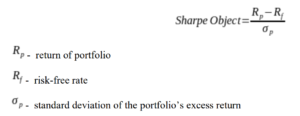A risk-adjusted return is a measure of an investment's profit or potential profit based on the level of risk that must be undertaken to obtain it. When comparing the returns of two assets or analyzing the returns of two portfolios, you should take into account not only the returns created by the investments but also the level of risk required to obtain these returns.

A risk-adjusted return might assist you in understanding what risk is being taken to achieve certain profits. It's a method for calculating an investment's return by looking at how much risk is taken to get the return.

By calculating the return an investment has produced over time to the amount of risk it has represented. If two or more investments provide the same return over the same time period, the one with the lowest risk has a higher risk-adjusted return.

## How risk-adjusted return ratios are calculated?

The most common way to calculate risk-adjusted return ratios is by using the Sharpe ratio. However, there are also other ways of effectively measuring risk, and assessing it.

### Sharpe ratio

The Sharpe ratio is a metric that quantifies how much return an investor receives, based on the amount of risk he takes on. The Sharpe ratio works by subtracting the asset's return from the returns that might have been obtained from a risk-free instrument such as treasury bills. Dividing that figure by the asset's standard deviation. The greater the ratio, the better you are compensated for the risk you are incurring.## Beta

Beta is a measure of the correlation between a particular investment and an index. It allows investors to understand whether a particular stock for instance is moving with the market or against it. It is extremely useful to analyze the volatility of that particular investment. Although volatility is not risk, beta can be an important way to understand how a particular investment should behave. Beta varies from 0 to 2.

A beta of 1 means that the stock and a particular index are moving perfectly together. So the stock will have the same expected level of volatility as the market. On the other hand, if the stock has a beta above 1, it means that it will be far more volatile. If the beta is lower than 1, then the stock and the index are moving in opposite directions.

## Why are risk-adjusted return ratios important?

Risk is defined as deviation from a predicted result. It might be represented positively or negatively in relation to a market benchmark. If an investor intends to earn high long-term returns on their investment, they must be willing to lose money in the short term. The amount of risk they are willing to take is determined by the investor's risk tolerance and risk capacity.

The risk-adjusted return quantifies how much risk is involved with generating a certain profit. The idea is used to compare the returns of various investments with varying risk levels associated with each one.

## Bottom line

Investors sometimes put too much emphasis on the returns they have generated. Without taking into consideration the risk they had to bear in order to achieve that. Risk-adjusted return ends up being a much better way of assessing how your investments have performed.

The fact that it takes into account the amount of risk you have undertaken, allows you to put things into perspective. Perhaps one investment or trading strategy that involves a high degree of risk, even if it generates a high rate of returns might not be the best approach.

Risk is perhaps one of the most important factors when it comes to investing and trading. Thus, overlooking it can be extremely detrimental to your portfolio.